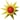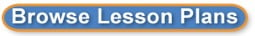Search results for linear equations:
 Browse All Lesson Plans Lesson Plan Name Grades Solving Systems of Linear Equations Using Substitution 7 to 12(0 stars, 1 ratings)This Algebra I lesson uses SMART Board technology to provide students with a physical sense of the process of substitution and how it can be used in solving a system of linear equations. Parallel and Perpendicular lines in our world 9 to 11(0 stars, 1 ratings)The students will collect real world examples of parallel and perpendicular lines and take pictures of them. Then the students will add a coordinate grid to the pictures and calculate the equations of the lines. Finally, the students will put together a presentation of their pictures, equations, and explanation of how the lines are related. "50 Ways to Use Your FlipCam" 9 to 12(0 stars, 1 ratings)This lesson/power point was developed in order to teach the audience (teachers/instructors) simple and quick ways to enhance their teaching and to help invest their student in their education by using a FlipCam. How Much does it Cost Project 7 to 9(0 stars, 2 ratings)In this project, students investigate pricing schemes for various entry fees and determine which is the better option depending on their situation. Interpret the equation 8 to 8(0 stars, 1 ratings)To interpret the equation of line, students will rotate through three stations. Each station will require the students to interpret the equation but using different techniques. Linear Relationships in the Real World 9 to 12(0 stars, 1 ratings)The student will use problem solving, mathematical communication, mathematical reasoning, connections and representations to solve multi-step equations in one variable with the variable on one of two sides of the equations while identifying at least 3 careers which utilize this skill. Paper Airplanes 9 to 12(0 stars, 2 ratings)Applications of Scatter Plots, Lines of Best Fit, Dependant & Independent Variables through the making and flying of paper airplanes. I have the class research several designs of airplanes, we discuss aerodynamics, construct a their desired model, fly the model, gather information and plot the results. Podcasting Parabolas 7 to 12(0 stars, 1 ratings)After an introductory lesson on parabolas, students will research parabolas, the general equation of a parabola from three points and photograph pictures of parabolas found in everyday life. Students will then organize the data to create and publish a podcast to be share with their peers in the classroom, as well as, around the world. (This is a 3-day lesson for the block schedule) Real World Equations! 9 to 12(0 stars, 1 ratings)Students will use digital cameras to take photos of items or situations that can be modeled using algebraic equations. Stations: Equation of a line and Slope 8 to 8(0 stars, 1 ratings)This is a stations lesson to check the learner's understanding of graphing lines and slope. The learner will receive immediate feedback at each station to determine if they fully understand the concept.